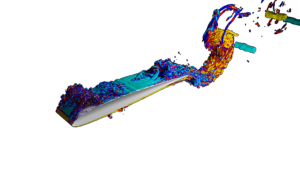# Snapshot: Hybrid Discontinuous Galerkin / Finite Volume (DG/FV) simulation of the multicomponent Shock-Bubble Interaction Problem

Simulation of the Shock-Bubble Interaction Problem with a hybrid entropy-stable DG/FV method and Adaptive Mesh Refinement (AMR) using Trixi (https://github.com/trixi-framework/Tr…).

The simulation uses a multicomponent model and computes the spatial operator as a blend of a first-order subcell FV method and a fourth-order DG scheme on a squared domain.

# New paper published: A Novel Robust Strategy for Discontinuous Galerkin Methods in Computational Fluid Mechanics: Why? When? What? Where?

In this paper we will review a recent emerging paradigm shift in the construction and analysis of high order Discontinuous Galerkin (DG) methods applied to approximate solutions of hyperbolic or mixed hyperbolic-parabolic partial differential equations (PDEs) in computational physics. There is a long history using DG methods to approximate the solution of PDEs in computational physics with successful applications in linear wave propagation, like those governed by Maxwell’s equations, incompressible and compressible fluid and plasma dynamics governed by the Navier-Stokes and the Magnetohydrodynamics equations, or as a solver for ordinary differential equations (ODEs), e.g., in structural mechanics. The DG method amalgamates ideas from several existing methods such as the Finite Element Galerkin method (FEM) and the Finite Volume method (FVM) and is specifically applied to problems with advection dominated properties, such as fast moving fluids or wave propagation. In the numerics community, DG methods are infamous for being computationally complex and, due to their high order nature, as having issues with robustness, i.e., these methods are sometimes prone to crashing easily. In this article we will focus on efficient nodal versions of the DG scheme and present recent ideas to restore its robustness, its connections to and influence by other sectors of the numerical community, such as the finite difference community, and further discuss this young, but rapidly developing research topic by highlighting the main contributions and a closing discussion about possible next lines of research.

Front. Phys. | doi: 10.3389/fphy.2020.500690

# New paper submitted: A Split-Form, Stable CG/DG-SEM for Wave Propagation Modeled by Linear Hyperbolic Systems

We present a hybrid continuous and discontinuous Galerkin spectral element approximation that leverages the advantages of each approach. The continuous Galerkin approximation is used on interior element faces where the equation properties are continuous. A discontinuous Galerkin approximation is used at physical boundaries and if there is a jump in properties at a face. The approximation uses a split form of the equations and two-point fluxes to ensure stability for unstructured quadrilateral/hexahedral meshes with curved elements. The approximation is also conservative and constant state preserving on such meshes. Spectral accuracy is obtained for all examples, which include wave scattering at a discontinuous medium boundary.

Preprint available at: arXiv:2012.06510

# New paper submitted: Stability of Discontinuous Galerkin Spectral Element Schemes for Wave Propagation when the Coefficient Matrices have Jumps

We use the behavior of the L2 norm of the solutions of linear hyperbolic equations with discontinuous coefficient matrices as a surrogate to infer stability of discontinuous Galerkin spectral element methods (DGSEM). Although the L2 norm is not bounded by the initial data for homogeneous and dissipative boundary conditions for such systems, the L2 norm is easier to work with than a norm that discounts growth due to the discontinuities. We show that the DGSEM with an upwind numerical flux that satisfies the Rankine-Hugoniot (or conservation) condition has the same energy bound as the partial differential equation does in the L2 norm, plus an added dissipation that depends on how much the approximate solution fails to satisfy the Rankine-Hugoniot jump.

Preprint available at arXiv:2011.11746

# New paper submitted: A Sub-Element Adaptive Shock Capturing Approach for Discontinuous Galerkin Methods

In this paper, a new strategy for a sub-element based shock capturing for discontinuous Galerkin (DG) approximations is presented. The idea is to interpret a DG element as a collection of data and construct a hierarchy of low to high order discretizations on this set of data, including a first order finite volume scheme up to the full order DG scheme. The different DG discretizations are then blended according to sub-element troubled cell indicators, resulting in a final discretization that adaptively blends from low to high order within a single DG element. The goal is to retain as much high order accuracy as possible, even in simulations with very strong shocks, as e.g. presented in the Sedov test. The framework retains the locality of the standard DG scheme and is hence well suited for a combination with adaptive mesh refinement and parallel computing. The numerical tests demonstrate the sub-element adaptive behavior of the new shock capturing approach and its high accuracy.

Preprint available at arXiv:2011.03338.

# Snapshot: 2D Riemann problem computed with a hybrid Discontinuous Galerkin / Finite Volume scheme

We present a 2D Riemann problem (configuration 3) computed with a hybrid Discontinuous Galerkin / Finite Volume scheme on a uniform grid with 1024^2 degrees of freedom.

In this hybrid approach we are blending an 8th order, 4th order and 2nd order DG as well as a 1st order FV scheme.

# Snapshot: A new approach for approximating conservation laws

A new approach for approximating conservation laws has been tested: instead of monitoring the changes of the means of a quantity within a certain volume over time like a finite volume procedure, this method mimics the behavior of simple solutions.

First the current state is split into waves w, each aligned along one of the eigenvectors of the Fluxjacobian and living on its own grid. Then the corresponding grids are being moved with their eigenvelocity and finally all waves w are being overlayed and normalized yielding the solution after one timestep.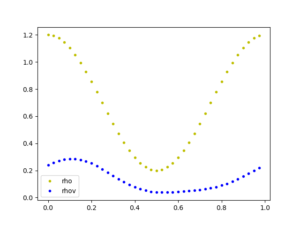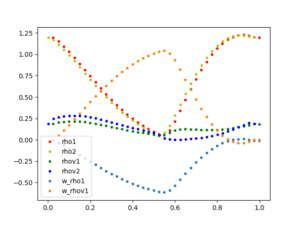The plots show the solution of isothermal Euler equations at Time T=0.0000 (initial condition) and T=0.0025 with N=40 gridpoints for each mesh.

# Snapshot: Higher-order schemes for the MHD equations

A robust and easy way of simulating a hyperbolic test case with discontinuities is to use a 1st order finite volume scheme. Using such a method for magnetohydrodynamics (MHD) problems like the Orszag-Tang vortex leads to following results: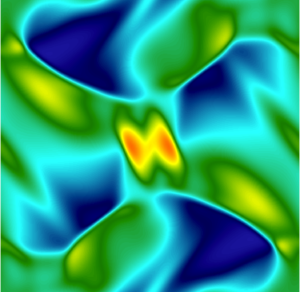For this and the following examples we used a 4th order time integration scheme and 256 degrees of freedom in each spatial direction.

A way to generate more accurate results is to increase the order of the scheme, which has to be treated with caution near discontinuities because oscillations may occur. To overcome this issue one could use higher order schemes in smooth regions and lower order schemes in regions with discontinuities. An example for such an approach is our so called DGFV scheme, which blends e.g. a 4th order Discontinuous Galerkin scheme with a 1st order Finite Volume scheme.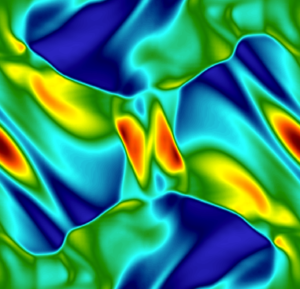Another way of doing it, is to use a suitable 4th order finite-volume scheme for MHD with a fitting limiter.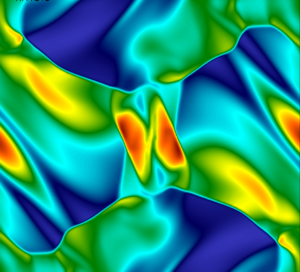# New paper published: Entropy-Stable p-Nonconforming Discretizations with the Summation-by-Parts Property for the Compressible Navier–Stokes Equations

The entropy-conservative/stable, curvilinear, nonconforming, p-refinement algorithm for hyperbolic conservation laws of Del Rey Fernández et al. (2019) is extended from the compressible Euler equations to the compressible Navier–Stokes equations. A simple and flexible coupling procedure with planar interpolation operators between adjoining nonconforming elements is used. Curvilinear volume metric terms are numerically approximated via a minimization procedure and satisfy the discrete geometric conservation law conditions. Distinct curvilinear surface metrics are used on the adjoining interfaces to construct the interface coupling terms, thereby localizing the discrete geometric conservation law constraints to each individual element. The resulting scheme is entropy conservative/stable, element-wise conservative, and freestream preserving. Viscous interface dissipation operators that retain the entropy stability of the base scheme are developed. The accuracy and stability of the resulting numerical scheme are shown to be comparable to those of the original conforming scheme in Carpenter et al. (2014) and Parsani et al. (2016), i.e., this scheme achieves ~p+1/2 convergence on geometrically high-order distorted element grids; this is demonstrated in the context of the viscous shock problem, the Taylor–Green vortex problem at a Reynolds number of Re = 1,600 and a subsonic turbulent flow past a sphere at Re = 2,000.

# New article published in ECCOMAS Newsletter (p.16-20): Split Form Discontinuous Galerkin Methods For Implicit Large Eddy Simulation Of Compressible Turbulence

As a teaser we show a numerical demonstration of the capabilities of the split form DG approach. We consider the flow past a plunging SD7003 airfoil at Mach number and cord length based Reynolds number Rec = 40,000. We subdivide the computational domain into 58,490 unstructured curvilinear hexahedral elements and use a polynomial degree N = 7 resulting in a total of about 150 million degrees of freedom.